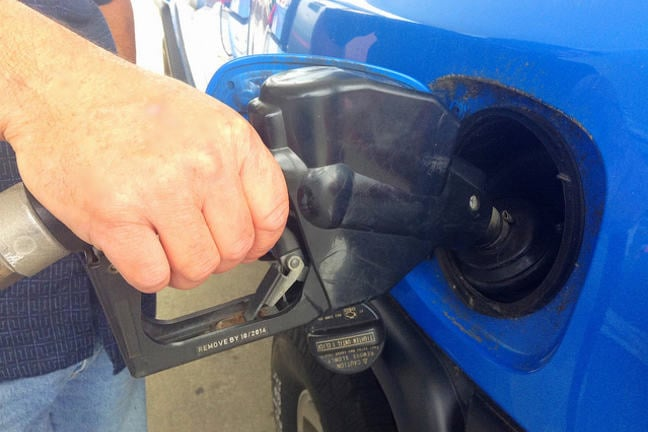## Want to keep learning?

This content is taken from the UNSW Sydney's online course, Maths for Humans: Linear, Quadratic & Inverse Relations. Join the course to learn more.
4.10

## UNSW SydneyWe all need it

# Gas stations and population

The larger a city is, the more gas stations it will have. On average, there ought to be some kind of quantitative relationship between the number of gas stations $$\normalsize{G}$$, and the population $$\normalsize{P}$$ of a city or state. This kind of information is potentially important to companies trying to decide if a given neighbourhood, city, or region should be considered for additional outlets.

In this step we will look at an unusual power law that connects the number of gas stations to population.

## Things are not always linear

One might expect that if a city doubles in size, the number of gas stations it needs also doubles. But this turns out not to be the case: rather a kind of increased efficiency arises, and the number of gas stations increases only by about $$\normalsize{70\%}$$. The relation between gas stations $$\normalsize{G}$$ and population $$\normalsize{P}$$ has been found to be something like

$\Large{G=A \times P^{0.77}} \tag{1} \label{1}$

for some constant $$\normalsize A$$. This explains the above observation about doubling the number of gas stations, since $$\normalsize{2^{0.77} \approxeq 1.705}$$.

But many questions arise. What is a good value for the constant $$\normalsize A$$, and does it depend on what region we are in? Does the law work not just for cities, but states/provinces as well? What about entire countries?

## Petrol stations in your town

The town of Taree in New South Wales, Australia, has 12 petrol stations (in Australia gas stations are called petrol stations) and a population of 49,000. That works out to be about one gas station for every 4000 inhabitants.

We can use this data in formula $$(\ref{1})$$ to find that

$\Large{12=A \times (49000)^{0.77}=4087 A}$

which gives $$\normalsize A \approx 0.003$$. Now that is assuming that the law $$(\ref{1})$$ holds with the exponent $$\normalsize 0.77$$, which is a big assumption. In fact it might be different depending on the town.

The power law relation $$(\ref{1})$$ holds precisely when a linear relationship holds between $$\normalsize \ln(P)$$ and $$\normalsize \ln(G)$$. So we can check if the exponent $$\normalsize 0.77$$ is appropriate by plotting $$\normalsize [\ln(P),\ln(G)]$$ for a few known data points.

## Discussion

What is the population $$\normalsize P$$ of your city and how many gas/petrol stations $$\normalsize G$$ are there? If we fit this into the simple model given by $$(\ref{1})$$, what is your value of $$\normalsize A$$?

If you have some more energy, please calculate the pair $$\normalsize [\ln(P), \ln(G)]$$ and tell us what it is.

Then let’s see if we can collectively plot these data points (which will be essentially what we call a log-log graph) and make some conclusions about the correct form of a power law. Is the power of $$\normalsize 0.77$$ accurate? Or has petrol consumption changed?

And then we can collectively publish a paper in the renowned Journal of Petrol, Population, and Power Laws!